# 逻辑门展区II

(4位元) 全加器

### 异或非门和异或门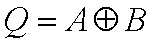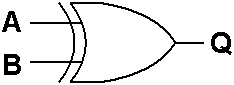ABQ
000
011
101
110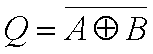ABQ
001
010
100
111

### 半加器和基本 (1位元) 全加器

 1 ←P +) 1 ←Q 1 0 ↑ ↑ 进位 和

 0 + 0 = 0 0 + 1 = 1 1 + 0 = 1 1 + 1 = 0 进位为1

PQCOS
0000
0101
1001
1110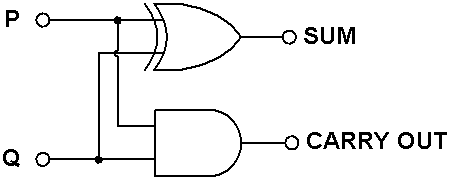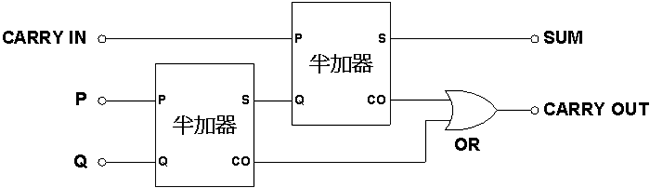### (4位元) 全加器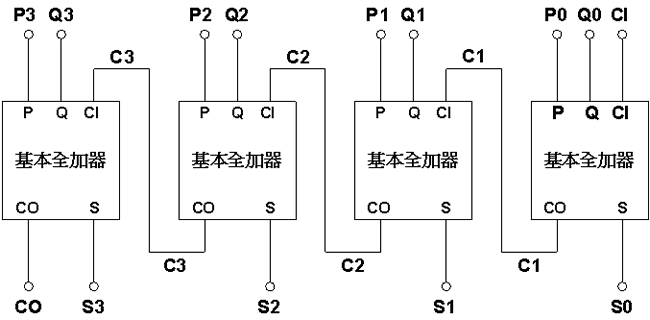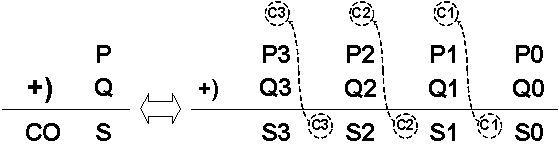P0 = 20 = 1 P1 = 21 = 2 P2 = 22 = 4 P3 = 23 = 8 P4 = 24 = 16

P10 = P4 x 24 + P3 x 23 + P2 x 22 + P1 x 21 + P0 x 20